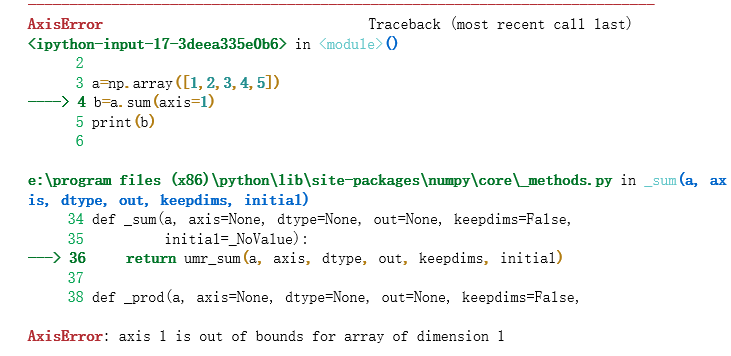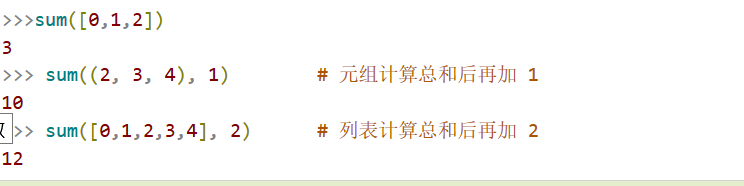• ## pythonsum函数的用法

千次阅读 2018-12-27 10:40:20
需要用到python sum（）函数，总结了一下，不对之处烦请指出，谢谢。 1维情况下  （1）输入axis=0 import numpy as np a=np.array([1,2,3,4,5]) b=a.sum(axis=0) print(b)  输出结果：15, 也就是一维数组...
需要用到python sum（）函数，总结了一下，不对之处烦请指出，谢谢。

1维情况下

（1）输入axis=0

import numpy as np

a=np.array([1,2,3,4,5])
b=a.sum(axis=0)
print(b)

输出结果：15,   也就是一维数组各个元素的和

（2）如果在一维情况下输入axis=1则会报如下错误，也就相当于数组维度越界。2维情况下

(1)输入axis=0

import numpy as np

b=np.array([[3,7,6],[2,4,5]])
print(b.sum(axis=0))

相当于是：
[[3,7,6],
[2,4,5]]
没一列对应元素相加
3+2,7+4,6+5
输出：
[ 5 11 11]

此时相当于是将每一列对应的元素相加，即3+2=5,7+4=11,6+5=11

（2）输入axis=1

import numpy as np

b=np.array([[3,7,6],[2,4,5]])
print(b.sum(axis=1))

输出为
[16 11]
相当于是3+7+6，2+4+5，即每一行的元素分别相加

相当于是3+7+6，2+4+5，即每一行的元素分别相加

3维情况下

（1）axis=0

import numpy as np

b=np.array([
[[3,7,6],[3,4,5]],
[[2,4,5],[1,1,1]]
])
print(b.sum(axis=0))
输出[[ 5 11 11]
[ 4  5  6]]
类似二维的情况[[3+2,7+4,6+5],[3+1,4+1,5+1]]

(2)axis=1

import numpy as np

b=np.array([
[[3,7,6],[3,4,5]],
[[2,4,5],[1,1,1]]
])
print(b.sum(axis=1))

输出[[ 6 11 11]
[ 3  5  6]]
三维中包含两行两列的二维数组，每一行对应的元素分别相加【[3+3,7+4,6+5],[2+1,4+1,5+1]】
以结果的第一行为例，结果由来是：
[
[ b+b, b+b, b+b ],
[ b+b, b+b, b+b ]
]

(3)axis=2

import numpy as np

b=np.array([
[[3,7,6],[3,4,5]],
[[2,4,5],[1,1,1]]
])
print(b.sum(axis=2))

输出[[16 12] ------>3+7+6,3+4+5
[11  3]------>2+4+5，1+1+1]



展开全文• numpy.sum numpy.sum(a,axis=None,dtype=None,out=None,keepdims=False)[source] Sum of array elements over a given axis. Parameters: a: array_like Elements to sum. axis: None or int ...
numpy.sum
numpy.sum(a, axis=None, dtype=None, out=None, keepdims=False)[source]
Sum of array elements over a given axis.
Parameters:

a : array_like

Elements to sum.

axis : None or int or tuple of ints, optional

Axis or axes along which a sum is performed. The default (axis = None) is perform a sum over all the dimensions of the input array. axis may be negative, in which case it counts from the last to the first axis.

New in version 1.7.0.

If this is a tuple of ints, a sum is performed on multiple axes, instead of a single axis or all the axes as before.

dtype : dtype, optional

The type of the returned array and of the accumulator in which the elements are summed. By default, the dtype of a is used. An exception is when a has an integer type with less precision than the default platform integer. In that case, the default platform integer is used instead.

out : ndarray, optional

Array into which the output is placed. By default, a new array is created. If out is given, it must be of the appropriate shape (the shape of a with axis removed, i.e., numpy.delete(a.shape, axis)). Its type is preserved. See doc.ufuncs (Section “Output arguments”) for more details.

keepdims : bool, optional

If this is set to True, the axes which are reduced are left in the result as dimensions with size one. With this option, the result will broadcast correctly against the original arr.

Returns:

sum_along_axis : ndarray

An array with the same shape as a, with the specified axis removed. If a is a 0-d array, or if axis is None, a scalar is returned. If an output array is specified, a reference to out is returned.

See also
ndarray.sumEquivalent method.cumsumCumulative sum of array elements.trapzIntegration of array values using the composite trapezoidal rule.mean, average

Notes
Arithmetic is modular when using integer types, and no error is raised on overflow.
Examples

>>>
>>> np.sum([0.5, 1.5])
2.0
>>> np.sum([0.5, 0.7, 0.2, 1.5], dtype=np.int32)
1
>>> np.sum([[0, 1], [0, 5]])
6
>>> np.sum([[0, 1], [0, 5]], axis=0)    #axis=0是按列求和
array([0, 6])
>>> np.sum([[0, 1], [0, 5]], axis=1)    #axis=1 是按行求和
array([1, 5])

If the accumulator is too small, overflow occurs:

>>>
>>> np.ones(128, dtype=np.int8).sum(dtype=np.int8)
-128

转载于:https://www.cnblogs.com/100thMountain/p/4719488.html
展开全文• sum函数是对系列求和的函数 1.语法： sum(iterable[, start]) 2.参数 iterable -- 可迭代对象，如：列表、元组、集合。 start -- 指定相加的参数，如果没有设置这个值，默认为0。 3.实例 ...
sum函数是对系列求和的函数
1.语法：
sum(iterable[, start])
2.参数
iterable -- 可迭代对象，如：列表、元组、集合。
start -- 指定相加的参数，如果没有设置这个值，默认为0。

3.实例展开全文• answer+=sum(S.count(i) for i in J) S、J都是字符串 ...我明白这句的意义，理解能完成的功能，但是找不到这种（for写在sum函数写法）的出处，不理解的点是for写在这个位置为什么会影响前面S.count(i)
• 今天小编就为大家分享一篇基于Python中求和函数sum用法详解，具有很好的参考价值，希望对大家有所帮助。一起跟随小编过来看看吧
• #!/usr/bin/env python # -*- coding: utf-8 -*- ...# # sum 函数原型是 sum(iterable, start), 还可以给个初始值 print(sum([1, 2, 3], 10)) # 16 # 另外一个比较Hack一些的用法, 展开2层的嵌套列

#!/usr/bin/env python
# -*- coding: utf-8 -*-

# sum的最常见用法计算一个序列的累加和
print(sum([1, 2, 3]))
# 6

# # sum 函数原型是 sum(iterable, start), 还可以给个初始值
print(sum([1, 2, 3], 10))
# 16

# 另外一个比较Hack一些的用法, 展开2层的嵌套列表
iterable = [[1, 2], [3, 4], [5, 6], [7, 8]]
start = 
print(sum(iterable, start))
# [0, 1, 2, 3, 4, 5, 6, 7, 8]

# sum函数是和下面的过程是等效的
for element in iterable:
start += element
print(start)
# [0, 1, 2, 3, 4, 5, 6, 7, 8]

# 实际上sum可以看成reduce()的特殊情况
from functools import reduce
from operator import add
start = []
print(reduce(add, iterable, start))
# [1, 2, 3, 4, 5, 6, 7, 8]
展开全文• 例: 两数相加函数 sum_lambda = lambda x, y: x+y print(sum_lambda(4,6)) lambda语句中，冒号前是参数，可以有多个，用逗号隔开，冒号右边的返回值。 例：能否被3整除 nums = [2, 18, 9, 22, 17, 24, 8, 12, 27] ...
• Numpy中sum函数Python自带sum函数）的作用是对元素求和。 无参时，所有全加； axis=0，按列相加； axis=1，按行相加； 下边通过例子来说明其用法： #!/usr/bin/env python # -*- coding:utf-8 -*- from numpy...numpy
• ## Python 中求和函数sum用法

万次阅读 多人点赞 2017-03-25 21:47:34
Python 中求和函数 sum 用法今天在看《集体编程智慧》这本书的时候，看到一段Python代码，当时是百思不得其解，总觉得是书中排版出错了，后来去了解了一下sum用法，看了一些Python大神写的代码后才发现是自己浅薄...math
• sum()方法对系列进行求和计算。针对元组，列表。对字符串会报错 >>>sum([0,1,2]) 3 >>> sum((2, 3, 4), 1) # 元组计算总和后再加 1 10 >>> sum([0,1,2,3,4], 2) # 列表计算总和后再加...
• sum()是python中一个很实用的函数，但是要注意用法。 第一次看到sum()函数，我就试着以下用法，结果悲剧了： 其实，sum()函数参数是一个list，例如：
• ## python中sum函数详解

千次阅读 2019-09-11 14:48:38
python内置的sum函数 对象是列表，元组，集合，可以混合起来使用 >>>sum([1,2,3],2) 8 >>>sum((1,2,3)) 6 >>>sum({1,2,3}) 6 >>>sum(range(5)) 10 numpy中sum...
• 三、sum() 四、round() 五、divmod() 一、min() min()函数：括号内带数字集合，求集合的最小值 # 将括号内的数据集合可以是列表，也可以直接元组 二、max() max() 函数：括号内带数字集合，求集合的最大...
• 今天在《集体编程智慧》这本书的时候，看到一段Python代码，经过研究之后大概弄懂了这段代码的意思，保存一下。 from math import sqrt def sim_distance(perfs,person1,person2):  si={}  for item in ...
• sum函数多种用法 连接型 列表相加 >>> sum(([1, 2], ), []) [1, 2, 3] 元组相加类似 >>> sum(((1, 2), (5,9)), ()) (1, 2, 5, 9)  计算总和后再加 >>> sum((1, 5, 7), 10) 23 ...LeetCode
• lambda匿名函数的格式：冒号前是参数，可以有多个，用逗号隔开，冒号右边的为表达式。 例1：传入一个参数的lambda函数 ...def sum(x,y): return x+y 用lambda来实现： p = lambda x,y:x+y print(p(4,6)) ...
• 本文实例讲述了Python高阶函数、常用内置函数用法。分享给大家供大家参考，具体如下： 高阶函数： 允许将函数作为参数传入另一个函数； 允许返回一个函数。 #返回值为函数的函数 sum=lambda x,y:x+y sub=lambda x,y:...
• def sum(x,y): return x+y 用lambda来实现： p = lambda x,y:x+y print(p(1,2)) 2、传入一个参数的lambda函数 a=lambda x:x*x print(a(2)) # 注意：这里直接a(2)可以执行，但没有输出的，因为没有print 3、...lambda
• 本文实例讲述了Python高阶函数、常用内置函数用法。分享给大家供大家参考，具体如下： 高阶函数： 允许将函数作为参数传入另一个函数； 允许返回一个函数。 #返回值为函数的函数 sum=lambda x,y:x+y sub=lambda x...
• sum()方法对进行求和计算。 sum(iterable[, start]) iterable -- 可迭代对象，如：列表、元组、集合。...map()是 Python 内置的高阶函数，它接收一个函数和一个 list，并通过把函数依次作用在 lis...
• 内置函数 abs() divmod() input() open() staticmethod() all() enumerate() int() ord() str() any() eval() isinstance() pow() sum() basestrin...
• python中的lambda函数用法 例1:传入多个参数的lambda函数 def sum(x,y): return x+y 用lambda来实现： p = lambda x,y:x+y print(p(4,6)) 例2：传入一个参数的lambda函数 a=lambda x:x*x print(a(3)) ...
• ## Python中zip函数用法

万次阅读 2012-09-11 14:57:20
看openstack的代码，遇到一个内建函数zip不明白其意思： # Adjust the weights in the grid by the functions weight adjustment # and sum them up to get a final list of weights. adjusted_scores = [] ...list tuples c 任务
• lambda 为匿名函数，语法格式为： lambda 形参1,形参2,…:单行表达式或调用其他函数的代码 调用格式： 1、把匿名函数赋值给另外一个变量，这个变量就是一个函数 2、把这个变量名当函数名使用：变量名(实参1,实参2) ......

# pythonsum函数用法python 订阅